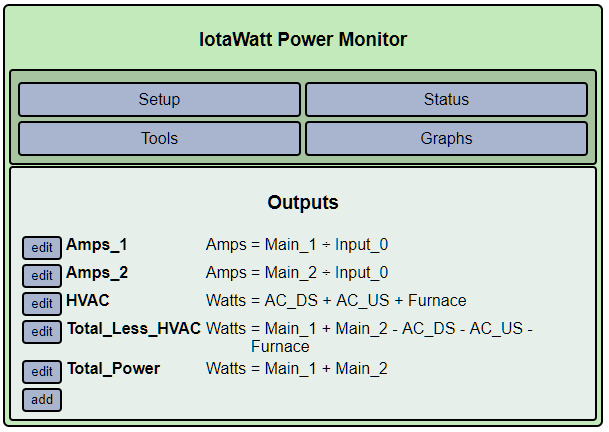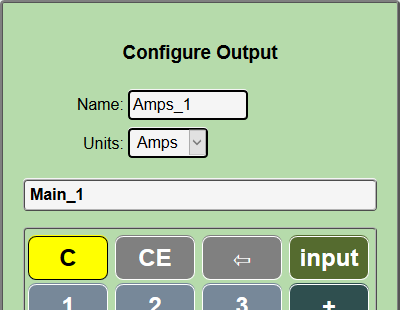# Using Outputs (Amps_1 = Main_1 / Input_0)

I added an Output with Amps = Main_1 / Input_0.

Here is what I see on the status screen:

Even taking the power factor into account, I could not figure out how Amps_1 in the status screen is calculated.

Here is my Output setup screen:It looks as if you are trying to get Amps by dividing Watts by voltage. That’s only valid when the power factor is 1.00 (real power = apparent power).

If you want to know the Amps for Main_1, remove the division:Simple as that.

Thanks! Just using Main_1 alone works.

I won’t try to figure out why the division doesn’t work. The pf is very close to one in my example but the current number using division is way too large (by 2x).

It has to do with using voltage in the formula. When you ask for amps, the value substituted for each input is the amps for that input. So you can, for instance, add the two mains to get total amps. But the script doesn’t know how to get amps from voltage (I don’t either), so it punts and returns something. I could figure it out, but it won’t help.

The important thing is that you can get all the common metrics about inputs by simply selecting the units and letting IoTaWatt figure out how to do it.